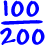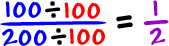# Simplifying Fractions

Page 1 of 3
Here's the game:
 We want to write fractions using the smallest numbers possible.

For example, look atCan you think of a better way to write this using smaller numbers? Let's see...

What if we divided the numerator and denominator by 100?Oh, yeah... This is a lot better!

Here are the two rules:

 1) You can divide the numerator and the denominator by any number... as long as you use the same number for both!  For example, you can't divide the numerator by 3 and the denominator by 5. 2) You need to get the numerator and denominator as small as possible.

Head on over to the next page to continue...